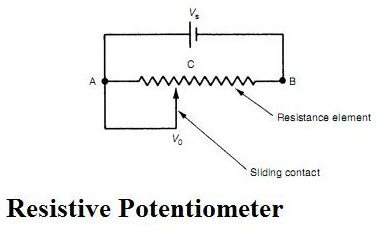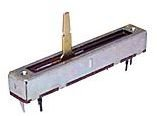# What is Potentiometer (Pots)? Potentiometer Transducers or Sensors.

## Principle of Working of Potentiometers

The potentiometer is also called as pots and it one of the most commonly used devise for measuring the displacement of the body. The potentiometer is the electrical type of transducer or sensor and it is of resistive type because it works on the principle of change of resistance of the wire with its length. The resistance of the wire is directly proportional to the length of the wire, thus as the length of the wire changes the resistance of the wire also changes.

## What is Potentiometer?

The potentiometer is an electric circuit in which the resistance can be changed manually by the sliding contacts. The typical potentiometer is shown in the figure below. Here the voltage Vs is applied across the two points of the wire A and B. C is the variable contact point between A and B and its position can be changed by the sliding contact. The voltage Vo is measured between the points A and C.

As per the resistance law of the conductor, the resistance of the conductor AC changes as the length of the wire AC changes. Accordingly the output voltage Vo between A and C also changes. The point C is the slider whose position is changed by the operator or by the motion of the body whose displacement is to be measured. The relationship between the length of the conductors and the voltage across them can be expressed as:

Vo/Vs = AC/AB## How Potentiometer is used as the Transducer

There are number of applications of the potentiometer or pots, of these one of the most common applications is the measurement of displacement. To measure the displacement of the body, this body, which is moving, is connected to the sliding element of the potentiometer.

As the body moves, the position of the slider located on the potentiometer also changes so the resistance between the fixed point and the slider changes. Due to this the voltage Vo across these points also changes. The change in voltage or the resistance is proportional to the change in the displacement of the body. Thus the voltage change indicates the displacement of the body. The potentiometer can be used for the measurement of translational as well as well rotational displacement. Since these potentiometers work on the principle of resistance, they are also called as the resistive potentiometers.## Important Performance Parameters of the Potentiometers

1) Potentiometer resolution: Though the resistance of the conductor is proportional to its length, when the slider moves over the wire the change in the resistance is not continuous function of the contact movement. The smallest increment of the slider that can be recorded across the whole length of the potentiometer is called as the resolution. If resistance winding is used in the potentiometer, its limiting resolution is measured as the reciprocal of the number of turns of the coil. The lower the length that can be recorded higher is the resolution of the potentiometer.

2) Potentiometer linearity: Ideally the resistance measured between the fixed point and the slider point is linear function of the contractor position relative to that end. In actual cases the change in resistance and the distance between the fixed and the moving point is never linear. The maximum allowable deviation between change in resistance and the linear position of the slider is called as the linearity tolerance.

## Reference

1) Book: Mechanical Measurements by Thomas G. Beckwith and N. Lewis Buck

2) Book: Measurement and Instrumentation Principles by Alan S Morris.

## Image Courtesy

1) Book: Measurement and Instrumentation Principles by Alan S Morris.

## This post is part of the series: What is Potentiometer?

This is the series of articles that describes what is potentiometer, also called as pots, its types and it can be used as the transducer.